## General Chemistry

The ideal gas laws show the correlation of the temperature, pressure, volume, and the amount of a gas. Although, this is not how the laws were determined, I found my students grasping the concepts a lot easier using the following model.

We are going to take a pump filled with some gas and a freely moving plunger and by changing the gas parameters determine their correlation.

# Boyle’s Law

Boyle’s Law shows the correlation of the pressure and the volume of a gas. To illustrate it, we change the volume of the gas by pushing down the plunger. This decreases the volume, and because the gas molecules have less space, the pressure is increasing:The observation is that the volume of a fixed quantity of gas at constant temperature is inversely proportional to its pressure.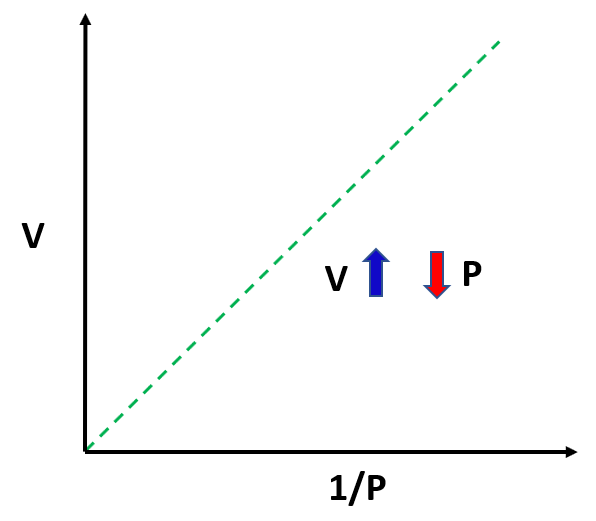This relationship can be written as:

${\rm{V}} \sim \,\frac{{\rm{1}}}{{\rm{P}}}$

To bring in the equal sign, we introduce a constant:

PV = constant

This can be explained using the example of a car dealership income. The income depends on the number of sales which we can represent as:

Income number of cars

However, we cannot say income = number of cars sold, so to switch an equal sign, we need to introduce a constant. This can be the price of the car transforming the equation to:

Income  = price x number of cars

So, for the gas pressure and volume, we are not interested too much in the constant, but rather in its linkage of pressure and volume at positions 1 and 2. Because the P x V product is constant, we can write that:

P1V1 = constant = P2V2

or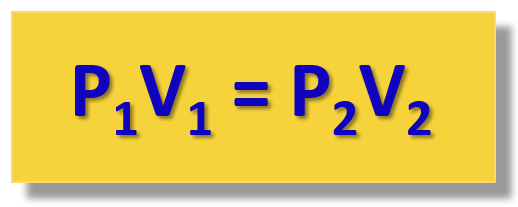This is the practical implication of the Boyle’s law that is used for solving gas problems.

For example,

The pressure of a gas is 2.30 atm in a 1.80 L container. Calculate the final pressure of the gas if the volume is decreased to 1.20 liters.

First, write down what you gave and what needs to be determined. If nothing is mentioned about any parameter, for example, the moles and the temperature in this case, it is assumed that they are constant, so you don’t need to worry about them.

P1 = 2.30 atm
V1 = 1.80 L
V2 = 1.20 L
P2 = ?

${{\rm{P}}_{\rm{1}}}{{\rm{V}}_{\rm{1}}}\; = \;{{\rm{P}}_{\rm{2}}}{{\rm{V}}_{\rm{2}}}$

Now, rearrange to calculate P2:

${{\rm{P}}_{\rm{2}}}\; = \;\frac{{{{\rm{P}}_{\rm{1}}}{{\rm{V}}_{\rm{1}}}}}{{{{\rm{V}}_{\rm{2}}}}}$

${{\rm{P}}_{\rm{2}}}\; = \;\frac{{{\rm{2}}{\rm{.30}}\;{\rm{atm}}\;{\rm{ \times 1}}{\rm{.80}}\;\cancel{{\rm{L}}}}}{{{\rm{1}}{\rm{.20}}\;\cancel{{\rm{L}}}}}\;{\rm{ = }}\;{\rm{3}}{\rm{.45}}\;{\rm{atm}}$

# Charle’s Law

Let’s now consider what happens if we heat up the gas leaving the plunger to move freely. The gas is going to expand, and the correlation is that the volume of a gas increases with temperature: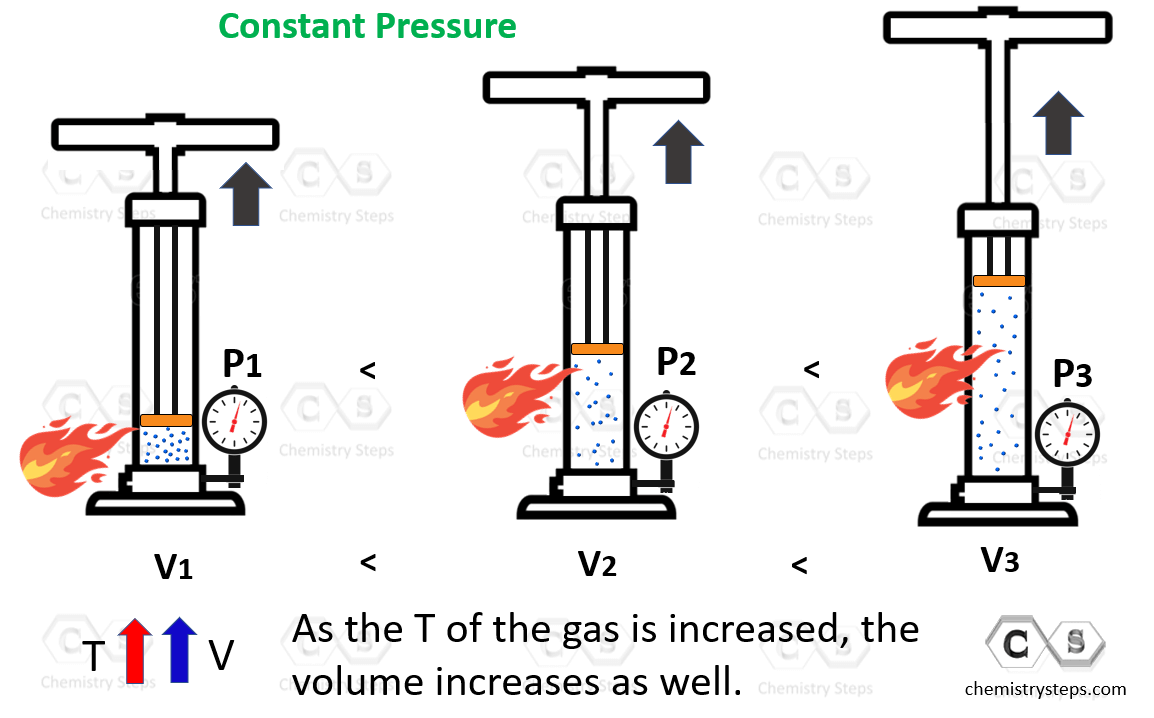The volume is directly proportional to the temperature of the gas: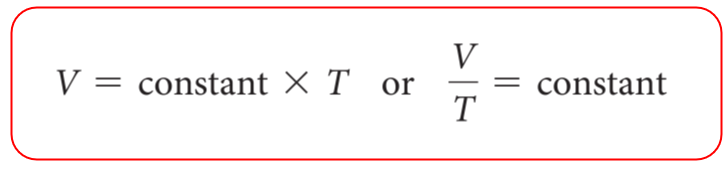Therefore, for different states of gas, we can write the Charle’s law as: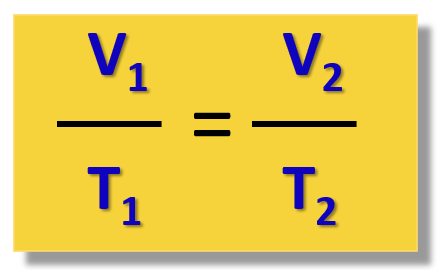For example,

What will be the final volume of a 3.50 L sample of nitrogen at 20 °C if it is heated to 200. °C?

Write down what is given and what needs to be determined first:

V1 = 3.50 L
T1 = 20 oC
T2 = 200. oC
V2 = ?

Now, before doing anything else, remember to always convert the temperature to Kelvin when solving a gas problem: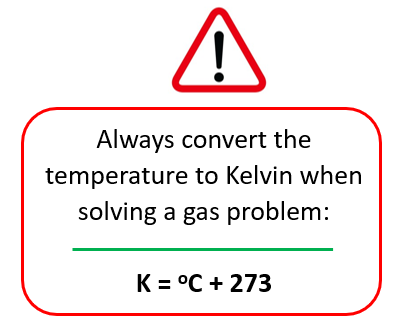So, T1 = 20 + 273 = 293 K,  T2 = 200 + 273 = 473 K

The question studies the correlation between the volume and the temperature of a gas, so we need to use the Charle’s law.

Write it down and rearrange it to get an expression of V2.

$\frac{{{{\rm{V}}_{\rm{1}}}}}{{{{\rm{T}}_{\rm{1}}}}}\; = \;\frac{{{{\rm{V}}_{\rm{2}}}}}{{{{\rm{T}}_{\rm{2}}}}}$

${{\rm{V}}_{\rm{2}}}\; = \;\frac{{{\rm{473}}\;\cancel{{\rm{K}}}\;{\rm{ \times }}\;{\rm{3}}{\rm{.50}}\;{\rm{L}}}}{{{\rm{293}}\;\cancel{{\rm{K}}}}}\;{\rm{ = }}\;{\rm{5}}{\rm{.65}}\;{\rm{L}}$

# Gay-Lussac’s law

To study the relationship between the pressure and the temperature of a gas, the barrel is held at a fixed position to prevent changing the volume, and the sample is heated up: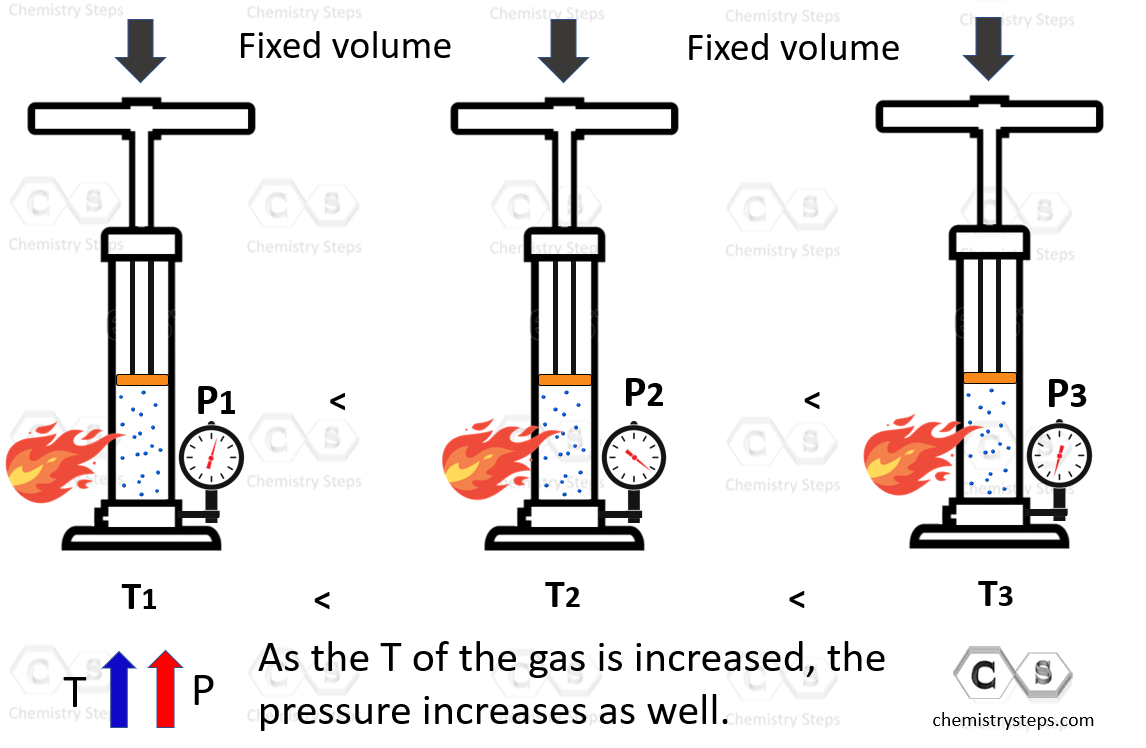In this case, the pressure increases with the temperature and for different states of the gas, the Gay-Lussac’s law is written as:For example,

A sample of helium gas at 1.40 atm is heated from 23.0 °C to 400.0 K. How many atmospheres is the final pressure of the helium gas?

P1 = 1.40 atm
T1 = 23.0 °C
P2 = 400.0 K
T2 = ?

Convert the temperature to Kelvin right away!

T1 = 23.0 + 273 = 296 K

Write down the gas law and rearrange it to get an expression of P2.

$\frac{{{{\rm{P}}_{\rm{1}}}}}{{{{\rm{T}}_{\rm{1}}}}}\; = \;\frac{{{{\rm{P}}_{\rm{2}}}}}{{{{\rm{T}}_{\rm{2}}}}}$

${{\rm{P}}_{\rm{2}}}\; = \;\frac{{{{\rm{P}}_{\rm{1}}}{{\rm{T}}_{\rm{2}}}}}{{{{\rm{T}}_{\rm{1}}}}}\; = \;\frac{{{\rm{1}}{\rm{.40}}\;{\rm{atm}}\;{\rm{ \times }}\;{\rm{400}}{\rm{.0}}\;\cancel{{\rm{K}}}}}{{{\rm{296}}\;\cancel{{\rm{K}}}}}\;{\rm{ = }}\;{\rm{1}}{\rm{.89}}\;{\rm{atm}}$

The Avogadro’s law of ideal gases demonstrates that the volume of a gas is directly proportional to its number of moles. In other words, the more gas, the larger the volume which again is a very intuitive observation.

For this, the plunger is again left free, and pumping some gas into the system increases the volume that the gas occupies:This is summarized in the following formula:Talking of the volume and the moles of a gas, remember that at STP, one mole of any gas occupies a volume of 22.4 L called the molar volume, Vo

This is because in ideal gases, the size of molecules is very small compared to the intermolecular distances. So, most of the volume is almost like an empty, and therefore, it does not matter what gas it is, the volume is determined to be 22.4 L at 0 oC and 1 atm.

# The Ideal Gas Law

The examples above are great to demonstrate the individual gas law, however, notice that in all experiments, we assumed or set up the experiment, such that two parameters are constant, and we study the correlation of the other two. For example, in the Boyle’s law, we study a constant amount of gas at a constant temperature and find that the pressure increase as the volume is decreased.

To combine all the laws together and have the four variables (n, P, V, T) in one place, the Ideal Gas Law equation is obtained: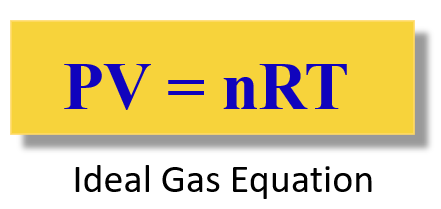The R is called the ideal gas constant. Although it has different values and units, you will mostly be using this:

$R\;{\rm{ = }}\;{\rm{0}}{\rm{.08206}}\;\frac{{{\rm{L}} \cdot {\rm{atm}}}}{{{\rm{mol}} \cdot {\rm{K}}}}$

The ideal gas law equation is used when you need to find P, V, T, or n, for a system where they do not change.

For example,

A sample of hydrogen gas is added into a 5.80 L container at 56.0 °C. How many moles of the gas is present in the container if the pressure is 6.70 atm?

Rearrange the ideal gas law to get an expression for the moles (n):

PV = nRT

${\rm{n}}\;{\rm{ = }}\;\frac{{{\rm{PV}}}}{{{\rm{RT}}}}$

${\rm{n}}\;{\rm{ = }}\;\frac{{{\rm{6}}{\rm{.70}}\;\cancel{{{\rm{atm}}}}\; \times \;{\rm{5}}{\rm{.80}}\;\cancel{{\rm{L}}}}}{{{\rm{0}}{\rm{.08206}}\;\cancel{{\rm{L}}}\;\cancel{{{\rm{atm}}}}\;\cancel{{{{\rm{K}}^{{\rm{ – 1}}}}}}\;{\rm{mo}}{{\rm{l}}^{{\rm{ – 1}}}}{\rm{ }} \times \;{\rm{329}}\;\cancel{{\rm{K}}}}}\; = \;1.44\;{\rm{mol}}$

Remember, to change the pressure to atm when the ideal gas law equation is used! This is because the units of R contain atm when the 0.08206 value is used. And this is what most problems in this chapter use.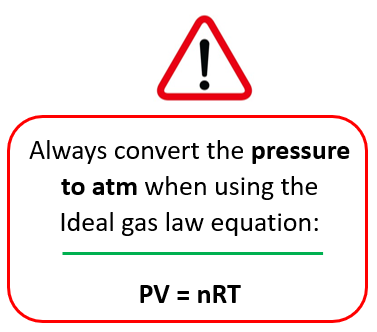## How do I know which gas law to use?

You are probably wondering about this question now that every gas law brings a new equation. For this, there is what is called the combined gas law and as long as you remember it, you do not need to remember all the gas laws to solve a problem.

Let’s keep it for another article because there is quite a lot of information in this one.

Check Also

#### Practice

1.

Boyle’s Law: The pressure of a gas is 2.30 atm in a 1.80 L container. Calculate the final pressure of the gas if the volume is decreased to 1.20 litters.

This content is available to registered users only. Click here to Register! By joining Chemistry Steps, you will gain instant access to the answers and solutions for all the Practice Problems and the powerful set of General Chemistry 1 and 2 Summary Study Guides.
Solution
This content is available to registered users only. Click here to Register! By joining Chemistry Steps, you will gain instant access to the answers and solutions for all the Practice Problems and the powerful set of General Chemistry 1 and 2 Summary Study Guides.
2.

Boyle’s Law: After changing the pressure of a gas sample from 760.0 torr to 0.800 atm, it occupies 4.30 L volume. What was the initial volume of the gas?

This content is available to registered users only. Click here to Register! By joining Chemistry Steps, you will gain instant access to the answers and solutions for all the Practice Problems and the powerful set of General Chemistry 1 and 2 Summary Study Guides.
3.

Charles’s Law: What will be the final volume of a 3.50 L sample of nitrogen at 20 °C if it is heated to 200 °C?

This content is available to registered users only. Click here to Register! By joining Chemistry Steps, you will gain instant access to the answers and solutions for all the Practice Problems and the powerful set of General Chemistry 1 and 2 Summary Study Guides.
Solution
This content is available to registered users only. Click here to Register! By joining Chemistry Steps, you will gain instant access to the answers and solutions for all the Practice Problems and the powerful set of General Chemistry 1 and 2 Summary Study Guides.
4.

Charles’s Law: The volume of a gas decreased from 2.40 L to 830. mL and the final temperature is set at 40.0 °C. Assuming a constant pressure, calculate the initial temperature of the gas in kelvins.